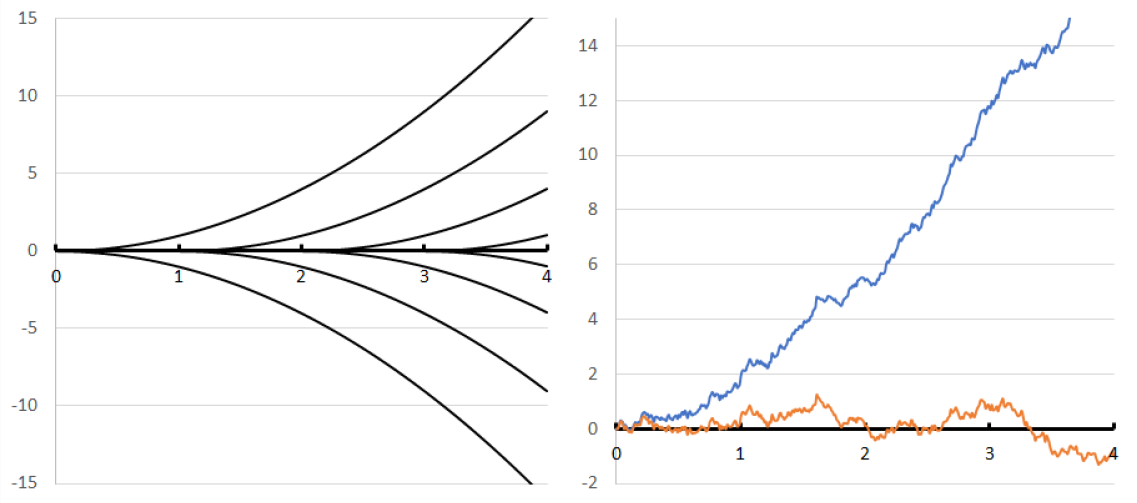Regularization by Noise for Stochastic Differential and Stochastic Partial Differential EquationsThe regularizing effects of noisy perturbations of differential equations is a central subject of stochastic analysis. Recent breakthroughs initiated a new wave of interest, particularly concerning non-Markovian, infinite dimensional, and rough-stochastic / Young-stochastic hybrid systems.

On the webinar, selected younger scholars will present their recent works on the topic.

When & Where:

• Wednesday, September 21st, 7:00 PT / 10:00 EST / 16:00 CET.
• Online, via Zoom. The registration form is available here.

Speakers:

• Helena Kremp, Freie Universität Berlin, Germany: Weak rough-path-type solutions for singular Lévy SDEs

Abstract: Since the works by Delarue, Diel and Cannizzaro, Chouk (in the Brownian noise setting), and our previous work, the existence and uniqueness of solutions to the martingale problem associated to multidimensional SDEs with additive $$\alpha$$-stable Lévy noise for $$\alpha$$ in (1, 2] and rough Besov drift of regularity $$\beta$$ > (2-2$$\alpha$$)/3 is known. Motivated by the equivalence of probabilistic weak solutions to SDEs with bounded, measurable drift and solutions to the martingale problem, we define a (non-canonical) weak solution concept for singular Lévy diffusions, proving moreover equivalence to the martingale solution in both the Young ($$\beta$$ > (1-$$\alpha$$)/2), as well as in the rough regime ($$\beta$$ > (2-2$$\alpha$$))/3). This turns out to be highly non-trivial in the rough case and forces us to define certain rough stochastic sewing integrals involved. In particular, we show that the canonical weak solution concept (introduced also by Athreya, Butkovsky, Mytnik), which is well-posed in the Young case, yields non-uniqueness of solutions in the rough case. If time permits, we apply our theory to the Brox diffusion with Lévy noise and prove a homogenization result for singular diffusions in the periodic Besov drift setting. The talk is based on joint work with Nicolas Perkowski.

• Lucio Galeati, University of Bonn, Germany: Advances on singular SDEs with fractional noise in subcritical regimes

Abstract: In recent years, there has been a lot of interest in regulariaation by noise for SDEs driven by fractional Brownian motion of parameter $$H\in (0,1)$$, with first results going back to Nualart, Ouknine (2002) and Catellier, Gubinelli (2016). The main challenges when dealing with these equations are the presence of a non-Lipschitz (possibly singular) drift and the lack of any martingale or Markovian structure; in particular, classical stochastic analysis and PDE tools break down for $$H\neq 1/2$$ and new techniques must be developed. Here I will present some advances on the topic, including generalizations to the regime $$H\in (1,\infty)$$, construction of the associated stochastic flow of solutions and stability estimates for SDEs driven by different drifts. The key tools in our analysis come from rough path theory and are given by Young integration and suitable versions of the stochastic sewing lemma. Based on a joint work (arXiv:2207.03475) with M. Gerencser (TU Wien).

• Lukas Anzeletti, Université Paris-Saclay, France: Regularisation by noise for SDEs with (fractional) Brownian noise and a comparison of different notions of solutions

Abstract: We study existence and uniqueness of solutions to the equation $$X_t=b(X_t)dt + dB_t$$, where $$b$$ may be distributional and $$B$$ is a fractional Brownian motion with Hurst parameter $$H\leq 1/2$$. We follow two approaches, namely using the stochastic sewing lemma and nonlinear Young integrals in $$p$$-variation. Furthermore, in the Brownian case $$H=1/2$$ we present examples of drifts in which the classical notion of a solution and solutions in a path-by-path sense coincide, respectively not coincide. Partly based on joint work with Alexandre Richard and Etienne Tanré.

• Igor Honoré, Université Claude Bernard Lyon 1, France: Selection by vanishing viscosity for the transport equation

Abstract: We consider a transport equation whose the coefficients can be in a negative Besov space, we provide controls in Hölder spaces of some kind of vanishing viscosity solutions from Kolmogorov equation associated with a stochastic process. We perform a new analysis based on a proxy around the flow associated with regularize coefficients, and thanks to a cut locus technique we obtain a Hölder control which does not depend on the regularity of the coefficients. The regularity only matters for the considered type of solution. As a consequence, we can give a meaning of some product of distributions. Finally, by a fixed point theorem, we can match the coefficient with the solution itself. This is exactly the inviscid Burgers’ equation whose the selection principle allows to avoid the well-known time singularity.

Discussant: Oleg Butkovsky, Weierstrass Institute for Applied Analysis and Stochastics, Germany

The webinar is part of YoungStatS project of the Young Statisticians Europe initiative (FENStatS) supported by the Bernoulli Society for Mathematical Statistics and Probability and the Institute of Mathematical Statistics (IMS).

If you missed this webinar, you can watch the recording on our YouTube channel.